# 神经网络变得轻松（第七部分）：自适应优化方法

24 二月 2021, 09:39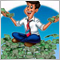0
495

### 1. 自适应优化方法的显著特征

#### 1.1. 自适应梯度方法（AdaGrad）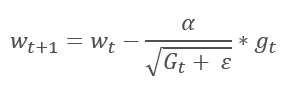#### 1.2. RMSProp 方法

AdaGrad 方法的逻辑延续自 RMSProp 方法。 为了避免学习率跌落到 0，在更新权重的公式分母中，已用梯度平方的指数均值替换过去的梯度平方和。 这种方法消除了分母中数值的恒定无限增长。 甚而，它更加关注表征模型当前状态的最新梯度值。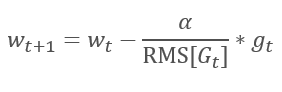#### 1.3. Adadelta 方法

Adadelta 自适应方法几乎与 RMSProp 同时提出。 此方法类似，它在更新权重的公式分母中采用了平方梯度总和的指数均值。 但与 RMSProp 不同，此方法彻底拒绝更新公式中的学习率，并在所分析参数中用之前修改的平方和的指数均值来替代。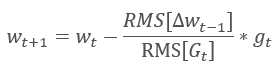#### 1.4. 自适应动量评估方法（亚当）

2014 年，Diederik P.Kingma 和 Jimmy Lei Ba 提出了自适应动量评估方法（Adam）。 根据作者的说法，该方法结合了 AdaGrad 和 RMSProp 方法的优点，非常适合在线训练。 该方法在不同样本上始终展现出良好的结果。 在各种软件包中，通常建议按照默认使用它。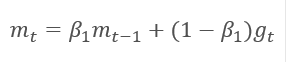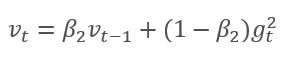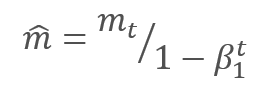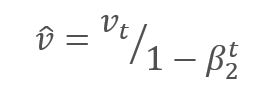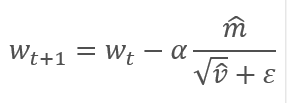### 2. 实现

#### 2.1. 构建 OpenCL 内核

• 权重矩阵 — matrix_w,
• 误差梯度矩阵  — matrix_g,
• 输入数据矩阵 — matrix_i,
• 梯度指数均值矩阵 — matrix_m,
• 平方梯度的指数均值矩阵   — matrix_v.

```__kernel void UpdateWeightsAdam(__global double *matrix_w,
__global double *matrix_g,
__global double *matrix_i,
__global double *matrix_m,
__global double *matrix_v,
int inputs, double l, double b1, double b2)

```

```  {
int i=get_global_id(0);
int j=get_global_id(1);
int wi=i*(inputs+1)+j*4;
```

```   double4 m, v, weight, inp;
switch(inputs-j*4)
{
case 0:
inp=(double4)(1,0,0,0);
weight=(double4)(matrix_w[wi],0,0,0);
m=(double4)(matrix_m[wi],0,0,0);
v=(double4)(matrix_v[wi],0,0,0);
break;
case 1:
inp=(double4)(matrix_i[j],1,0,0);
weight=(double4)(matrix_w[wi],matrix_w[wi+1],0,0);
m=(double4)(matrix_m[wi],matrix_m[wi+1],0,0);
v=(double4)(matrix_v[wi],matrix_v[wi+1],0,0);
break;
case 2:
inp=(double4)(matrix_i[j],matrix_i[j+1],1,0);
weight=(double4)(matrix_w[wi],matrix_w[wi+1],matrix_w[wi+2],0);
m=(double4)(matrix_m[wi],matrix_m[wi+1],matrix_m[wi+2],0);
v=(double4)(matrix_v[wi],matrix_v[wi+1],matrix_v[wi+2],0);
break;
case 3:
inp=(double4)(matrix_i[j],matrix_i[j+1],matrix_i[j+2],1);
weight=(double4)(matrix_w[wi],matrix_w[wi+1],matrix_w[wi+2],matrix_w[wi+3]);
m=(double4)(matrix_m[wi],matrix_m[wi+1],matrix_m[wi+2],matrix_m[wi+3]);
v=(double4)(matrix_v[wi],matrix_v[wi+1],matrix_v[wi+2],matrix_v[wi+3]);
break;
default:
inp=(double4)(matrix_i[j],matrix_i[j+1],matrix_i[j+2],matrix_i[j+3]);
weight=(double4)(matrix_w[wi],matrix_w[wi+1],matrix_w[wi+2],matrix_w[wi+3]);
m=(double4)(matrix_m[wi],matrix_m[wi+1],matrix_m[wi+2],matrix_m[wi+3]);
v=(double4)(matrix_v[wi],matrix_v[wi+1],matrix_v[wi+2],matrix_v[wi+3]);
break;
}
```

`   double4 g=matrix_g[i]*inp;`

```   double4 mt=b1*m+(1-b1)*g;
double4 vt=b2*v+(1-b2)*pow(g,2)+0.00000001;
```

```   double4 delta=l*mt/sqrt(vt);

```

```   switch(inputs-j*4)
{
case 2:
matrix_w[wi+2]+=delta.s2;
matrix_m[wi+2]=mt.s2;
matrix_v[wi+2]=vt.s2;
case 1:
matrix_w[wi+1]+=delta.s1;
matrix_m[wi+1]=mt.s1;
matrix_v[wi+1]=vt.s1;
case 0:
matrix_w[wi]+=delta.s0;
matrix_m[wi]=mt.s0;
matrix_v[wi]=vt.s0;
break;
default:
matrix_w[wi]+=delta.s0;
matrix_m[wi]=mt.s0;
matrix_v[wi]=vt.s0;
matrix_w[wi+1]+=delta.s1;
matrix_m[wi+1]=mt.s1;
matrix_v[wi+1]=vt.s1;
matrix_w[wi+2]+=delta.s2;
matrix_m[wi+2]=mt.s2;
matrix_v[wi+2]=vt.s2;
matrix_w[wi+3]+=delta.s3;
matrix_m[wi+3]=mt.s3;
matrix_v[wi+3]=vt.s3;
break;
}
};
```

#### 2.2. 修改主程序里的神经元类代码

```#define def_k_UpdateWeightsAdam   4
#define def_k_uwa_matrix_w        0
#define def_k_uwa_matrix_g        1
#define def_k_uwa_matrix_i        2
#define def_k_uwa_matrix_m        3
#define def_k_uwa_matrix_v        4
#define def_k_uwa_inputs          5
#define def_k_uwa_l               6
#define def_k_uwa_b1              7
#define def_k_uwa_b2              8
```

```enum ENUM_OPTIMIZATION
{
SGD,
ADAM
};
//---
enum ENUM_BUFFERS
{
WEIGHTS,
DELTA_WEIGHTS,
OUTPUT,
GRADIENT,
FIRST_MOMENTUM,
SECOND_MOMENTUM
};
```

```class CNeuronBaseOCL    :  public CObject
{
protected:
.........
.........
..........
CBufferDouble     *FirstMomentum;
CBufferDouble     *SecondMomentum;
//---
.........
.........
const double      b1;
const double      b2;
int               t;
//---
.........
.........
ENUM_OPTIMIZATION optimization;

```

```CNeuronBaseOCL::CNeuronBaseOCL(void)   :  alpha(momentum),
activation(TANH),
optimization(SGD),
b1(0.9),
b2(0.999),
t(1)
{
OpenCL=NULL;
Output=new CBufferDouble();
PrevOutput=new CBufferDouble();
Weights=new CBufferDouble();
DeltaWeights=new CBufferDouble();
Gradient=new CBufferDouble();
FirstMomentum=new CBufferDouble();
SecondMomentum=new CBufferDouble();
}

```

```CNeuronBaseOCL::~CNeuronBaseOCL(void)
{
if(CheckPointer(Output)!=POINTER_INVALID)
delete Output;
if(CheckPointer(PrevOutput)!=POINTER_INVALID)
delete PrevOutput;
if(CheckPointer(Weights)!=POINTER_INVALID)
delete Weights;
if(CheckPointer(DeltaWeights)!=POINTER_INVALID)
delete DeltaWeights;
if(CheckPointer(Gradient)!=POINTER_INVALID)
delete Gradient;
if(CheckPointer(FirstMomentum)!=POINTER_INVALID)
delete FirstMomentum;
if(CheckPointer(SecondMomentum)!=POINTER_INVALID)
delete SecondMomentum;
OpenCL=NULL;
}

```

```bool CNeuronBaseOCL::Init(uint numOutputs,uint myIndex,COpenCLMy *open_cl,uint numNeurons, ENUM_OPTIMIZATION optimization_type)
{
if(CheckPointer(open_cl)==POINTER_INVALID || numNeurons<=0)
return false;
OpenCL=open_cl;
optimization=optimization_type;
//---
....................
....................
....................
....................
//---
if(numOutputs>0)
{
if(CheckPointer(Weights)==POINTER_INVALID)
{
Weights=new CBufferDouble();
if(CheckPointer(Weights)==POINTER_INVALID)
return false;
}
int count=(int)((numNeurons+1)*numOutputs);
if(!Weights.Reserve(count))
return false;
for(int i=0;i<count;i++)
{
double weigh=(MathRand()+1)/32768.0-0.5;
if(weigh==0)
weigh=0.001;
if(!Weights.Add(weigh))
return false;
}
if(!Weights.BufferCreate(OpenCL))
return false;
//---
if(optimization==SGD)
{
if(CheckPointer(DeltaWeights)==POINTER_INVALID)
{
DeltaWeights=new CBufferDouble();
if(CheckPointer(DeltaWeights)==POINTER_INVALID)
return false;
}
if(!DeltaWeights.BufferInit(count,0))
return false;
if(!DeltaWeights.BufferCreate(OpenCL))
return false;
if(CheckPointer(FirstMomentum)==POINTER_INVALID)
delete FirstMomentum;
if(CheckPointer(SecondMomentum)==POINTER_INVALID)
delete SecondMomentum;
}
else
{
if(CheckPointer(DeltaWeights)==POINTER_INVALID)
delete DeltaWeights;
//---
if(CheckPointer(FirstMomentum)==POINTER_INVALID)
{
FirstMomentum=new CBufferDouble();
if(CheckPointer(FirstMomentum)==POINTER_INVALID)
return false;
}
if(!FirstMomentum.BufferInit(count,0))
return false;
if(!FirstMomentum.BufferCreate(OpenCL))
return false;
//---
if(CheckPointer(SecondMomentum)==POINTER_INVALID)
{
SecondMomentum=new CBufferDouble();
if(CheckPointer(SecondMomentum)==POINTER_INVALID)
return false;
}
if(!SecondMomentum.BufferInit(count,0))
return false;
if(!SecondMomentum.BufferCreate(OpenCL))
return false;
}
}
else
{
if(CheckPointer(Weights)!=POINTER_INVALID)
delete Weights;
if(CheckPointer(DeltaWeights)!=POINTER_INVALID)
delete DeltaWeights;
}
//---
return true;
}

```

```bool CNeuronBaseOCL::updateInputWeights(CNeuronBaseOCL *NeuronOCL)
{
if(CheckPointer(OpenCL)==POINTER_INVALID || CheckPointer(NeuronOCL)==POINTER_INVALID)
return false;
uint global_work_offset={0,0};
uint global_work_size;
global_work_size=Neurons();
global_work_size=NeuronOCL.Neurons();
if(optimization==SGD)
{

```

```      OpenCL.SetArgumentBuffer(def_k_UpdateWeightsMomentum,def_k_uwm_matrix_w,NeuronOCL.getWeightsIndex());
OpenCL.SetArgumentBuffer(def_k_UpdateWeightsMomentum,def_k_uwm_matrix_g,getGradientIndex());
OpenCL.SetArgumentBuffer(def_k_UpdateWeightsMomentum,def_k_uwm_matrix_i,NeuronOCL.getOutputIndex());
OpenCL.SetArgumentBuffer(def_k_UpdateWeightsMomentum,def_k_uwm_matrix_dw,NeuronOCL.getDeltaWeightsIndex());
OpenCL.SetArgument(def_k_UpdateWeightsMomentum,def_k_uwm_inputs,NeuronOCL.Neurons());
OpenCL.SetArgument(def_k_UpdateWeightsMomentum,def_k_uwm_learning_rates,eta);
OpenCL.SetArgument(def_k_UpdateWeightsMomentum,def_k_uwm_momentum,alpha);
ResetLastError();
if(!OpenCL.Execute(def_k_UpdateWeightsMomentum,2,global_work_offset,global_work_size))
{
printf("Error of execution kernel UpdateWeightsMomentum: %d",GetLastError());
return false;
}
}

```

```   else
{
if(!OpenCL.SetArgumentBuffer(def_k_UpdateWeightsAdam,def_k_uwa_matrix_w,NeuronOCL.getWeightsIndex()))
return false;
if(!OpenCL.SetArgumentBuffer(def_k_UpdateWeightsAdam,def_k_uwa_matrix_g,getGradientIndex()))
return false;
if(!OpenCL.SetArgumentBuffer(def_k_UpdateWeightsAdam,def_k_uwa_matrix_i,NeuronOCL.getOutputIndex()))
return false;
if(!OpenCL.SetArgumentBuffer(def_k_UpdateWeightsAdam,def_k_uwa_matrix_m,NeuronOCL.getFirstMomentumIndex()))
return false;
if(!OpenCL.SetArgumentBuffer(def_k_UpdateWeightsAdam,def_k_uwa_matrix_v,NeuronOCL.getSecondMomentumIndex()))
return false;

```

```      double lt=eta*sqrt(1-pow(b2,t))/(1-pow(b1,t));

```

```      if(!OpenCL.SetArgument(def_k_UpdateWeightsAdam,def_k_uwa_inputs,NeuronOCL.Neurons()))
return false;
if(!OpenCL.SetArgument(def_k_UpdateWeightsAdam,def_k_uwa_l,lt))
return false;
if(!OpenCL.SetArgument(def_k_UpdateWeightsAdam,def_k_uwa_b1,b1))
return false;
if(!OpenCL.SetArgument(def_k_UpdateWeightsAdam,def_k_uwa_b2,b2))
return false;

```

```      uint rest=global_work_size%4;
global_work_size=(global_work_size-rest)/4 + (rest>0 ? 1 : 0);

```

```      ResetLastError();
if(!OpenCL.Execute(def_k_UpdateWeightsAdam,2,global_work_offset,global_work_size))
{
printf("Error of execution kernel UpdateWeightsAdam: %d",GetLastError());
return false;
}
t++;
}

```

```//---
return NeuronOCL.Weights.BufferRead();
}
```

```bool CNeuronBaseOCL::Save(const int file_handle)
{
if(file_handle==INVALID_HANDLE)
return false;
if(FileWriteInteger(file_handle,Type())<INT_VALUE)
return false;
//---
if(FileWriteInteger(file_handle,(int)activation,INT_VALUE)<INT_VALUE)
return false;
if(FileWriteInteger(file_handle,(int)optimization,INT_VALUE)<INT_VALUE)
return false;
if(FileWriteInteger(file_handle,(int)t,INT_VALUE)<INT_VALUE)
return false;
```

```   if(CheckPointer(Output)==POINTER_INVALID || !Output.BufferRead() || !Output.Save(file_handle))
return false;
if(CheckPointer(PrevOutput)==POINTER_INVALID || !PrevOutput.BufferRead() || !PrevOutput.Save(file_handle))
return false;
if(CheckPointer(Gradient)==POINTER_INVALID || !Gradient.BufferRead() || !Gradient.Save(file_handle))
return false;
//---
if(CheckPointer(Weights)==POINTER_INVALID)
{
FileWriteInteger(file_handle,0);
return true;
}
else
FileWriteInteger(file_handle,1);
//---
if(CheckPointer(Weights)==POINTER_INVALID || !Weights.BufferRead() || !Weights.Save(file_handle))
return false;

```

```   if(optimization==SGD)
{
if(CheckPointer(DeltaWeights)==POINTER_INVALID || !DeltaWeights.BufferRead() || !DeltaWeights.Save(file_handle))
return false;
}
else
{
if(CheckPointer(FirstMomentum)==POINTER_INVALID || !FirstMomentum.BufferRead() || !FirstMomentum.Save(file_handle))
return false;
if(CheckPointer(SecondMomentum)==POINTER_INVALID || !SecondMomentum.BufferRead() || !SecondMomentum.Save(file_handle))
return false;
}
//---
return true;
}
```

#### 2.3. 修改不使用 OpenCL 的类代码

```class CConnection : public CObject
{
public:
double            weight;
double            deltaWeight;
double            mt;
double            vt;

CConnection(double w) { weight=w; deltaWeight=0; mt=0; vt=0; }
```

还必须将新变量的处理添加到保存和加载连接数据的方法当中。

```bool CConnection::Save(int file_handle)
{
...........
...........
...........
if(FileWriteDouble(file_handle,mt)<=0)
return false;
if(FileWriteDouble(file_handle,vt)<=0)
return false;
//---
return true;
}
//+------------------------------------------------------------------+
//|                                                                  |
//+------------------------------------------------------------------+
bool CConnection::Load(int file_handle)
{
............
............
............
mt=FileReadDouble(file_handle);
vt=FileReadDouble(file_handle);
//---
return true;
}
```

```class CNeuronBase    :  public CObject
{
protected:
.........
.........
.........
ENUM_OPTIMIZATION optimization;

const double      b1;
const double      b2;
int               t;
```

```bool CNeuronBase::Init(uint numOutputs,uint myIndex, ENUM_OPTIMIZATION optimization_type)
{
optimization=optimization_type;

```

```bool CNeuron::updateInputWeights(CLayer *&prevLayer)
{
if(CheckPointer(prevLayer)==POINTER_INVALID)
return false;
//---
double lt=eta*sqrt(1-pow(b2,t))/(1-pow(b1,t));
int total=prevLayer.Total();
for(int n=0; n<total && !IsStopped(); n++)
{
CNeuron *neuron= prevLayer.At(n);
CConnection *con=neuron.Connections.At(m_myIndex);
if(CheckPointer(con)==POINTER_INVALID)
continue;
if(optimization==SGD)
con.weight+=con.deltaWeight=(gradient!=0 ? eta*neuron.getOutputVal()*gradient : 0)+(con.deltaWeight!=0 ? alpha*con.deltaWeight : 0);
else
{
con.mt=b1*con.mt+(1-b1)*gradient;
con.vt=b2*con.vt+(1-b2)*pow(gradient,2)+0.00000001;
con.weight+=con.deltaWeight=lt*con.mt/sqrt(con.vt);
t++;
}
}
//---
return true;
}
```

#### 2.4. 修改主程序中的神经网络类代码

```class CLayerDescription    :  public CObject
{
public:
CLayerDescription(void);
~CLayerDescription(void) {};
//---
int               type;
int               count;
int               window;
int               step;
ENUM_ACTIVATION   activation;
ENUM_OPTIMIZATION optimization;
};
```

```CNet::CNet(CArrayObj *Description)
{
if(CheckPointer(Description)==POINTER_INVALID)
return;
//---
int total=Description.Total();
if(total<=0)
return;
//---
layers=new CArrayLayer();
if(CheckPointer(layers)==POINTER_INVALID)
return;
//---
CLayer *temp;
CLayerDescription *desc=NULL, *next=NULL, *prev=NULL;
CNeuronBase *neuron=NULL;
CNeuronProof *neuron_p=NULL;
int output_count=0;
int temp_count=0;
//---
next=Description.At(1);
if(next.type==defNeuron || next.type==defNeuronBaseOCL)
{
opencl=new COpenCLMy();
if(CheckPointer(opencl)!=POINTER_INVALID && !opencl.Initialize(cl_program,true))
delete opencl;
}
else
{
if(CheckPointer(opencl)!=POINTER_INVALID)
delete opencl;
}
//---
for(int i=0; i<total; i++)
{
prev=desc;
desc=Description.At(i);
if((i+1)<total)
{
next=Description.At(i+1);
if(CheckPointer(next)==POINTER_INVALID)
return;
}
else
next=NULL;
int outputs=(next==NULL || (next.type!=defNeuron && next.type!=defNeuronBaseOCL) ? 0 : next.count);
temp=new CLayer(outputs);
int neurons=(desc.count+(desc.type==defNeuron || desc.type==defNeuronBaseOCL ? 1 : 0));
if(CheckPointer(opencl)!=POINTER_INVALID)
{
CNeuronBaseOCL *neuron_ocl=NULL;
switch(desc.type)
{
case defNeuron:
case defNeuronBaseOCL:
neuron_ocl=new CNeuronBaseOCL();
if(CheckPointer(neuron_ocl)==POINTER_INVALID)
{
delete temp;
return;
}
if(!neuron_ocl.Init(outputs,0,opencl,desc.count,desc.optimization))
{
delete temp;
return;
}
neuron_ocl.SetActivationFunction(desc.activation);
if(!temp.Add(neuron_ocl))
{
delete neuron_ocl;
delete temp;
return;
}
neuron_ocl=NULL;
break;
default:
return;
break;
}
}
else
for(int n=0; n<neurons; n++)
{
switch(desc.type)
{
case defNeuron:
neuron=new CNeuron();
if(CheckPointer(neuron)==POINTER_INVALID)
{
delete temp;
delete layers;
return;
}
neuron.Init(outputs,n,desc.optimization);
neuron.SetActivationFunction(desc.activation);
break;
case defNeuronConv:
neuron_p=new CNeuronConv();
if(CheckPointer(neuron_p)==POINTER_INVALID)
{
delete temp;
delete layers;
return;
}
if(CheckPointer(prev)!=POINTER_INVALID)
{
if(prev.type==defNeuron)
{
temp_count=(int)((prev.count-desc.window)%desc.step);
output_count=(int)((prev.count-desc.window-temp_count)/desc.step+(temp_count==0 ? 1 : 2));
}
else
if(n==0)
{
temp_count=(int)((output_count-desc.window)%desc.step);
output_count=(int)((output_count-desc.window-temp_count)/desc.step+(temp_count==0 ? 1 : 2));
}
}
if(neuron_p.Init(outputs,n,desc.window,desc.step,output_count,desc.optimization))
neuron=neuron_p;
break;
case defNeuronProof:
neuron_p=new CNeuronProof();
if(CheckPointer(neuron_p)==POINTER_INVALID)
{
delete temp;
delete layers;
return;
}
if(CheckPointer(prev)!=POINTER_INVALID)
{
if(prev.type==defNeuron)
{
temp_count=(int)((prev.count-desc.window)%desc.step);
output_count=(int)((prev.count-desc.window-temp_count)/desc.step+(temp_count==0 ? 1 : 2));
}
else
if(n==0)
{
temp_count=(int)((output_count-desc.window)%desc.step);
output_count=(int)((output_count-desc.window-temp_count)/desc.step+(temp_count==0 ? 1 : 2));
}
}
if(neuron_p.Init(outputs,n,desc.window,desc.step,output_count,desc.optimization))
neuron=neuron_p;
break;
case defNeuronLSTM:
neuron_p=new CNeuronLSTM();
if(CheckPointer(neuron_p)==POINTER_INVALID)
{
delete temp;
delete layers;
return;
}
output_count=(next!=NULL ? next.window : desc.step);
if(neuron_p.Init(outputs,n,desc.window,1,output_count,desc.optimization))
neuron=neuron_p;
break;
}
if(!temp.Add(neuron))
{
delete temp;
delete layers;
return;
}
neuron=NULL;
}
if(!layers.Add(temp))
{
delete temp;
delete layers;
return;
}
}
//---
if(CheckPointer(opencl)==POINTER_INVALID)
return;
//--- create kernels
opencl.SetKernelsCount(5);
opencl.KernelCreate(def_k_FeedForward,"FeedForward");
opencl.KernelCreate(def_k_CaclOutputGradient,"CaclOutputGradient");
opencl.KernelCreate(def_k_CaclHiddenGradient,"CaclHiddenGradient");
opencl.KernelCreate(def_k_UpdateWeightsMomentum,"UpdateWeightsMomentum");
opencl.KernelCreate(def_k_UpdateWeightsAdam,"UpdateWeightsAdam");
//---
return;
}
```

### 3. 测试

```      desc.count=(int)HistoryBars*12;
desc.type=defNeuron;
desc.optimization=ADAM;

```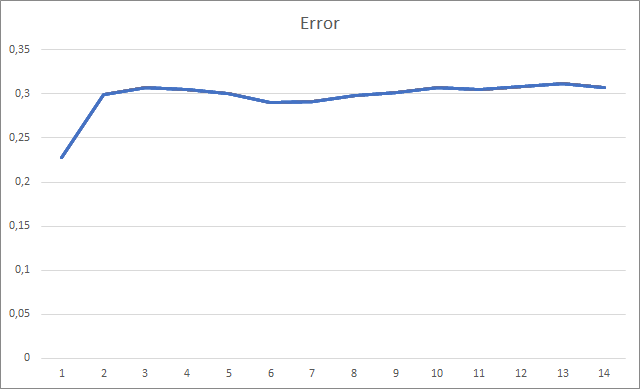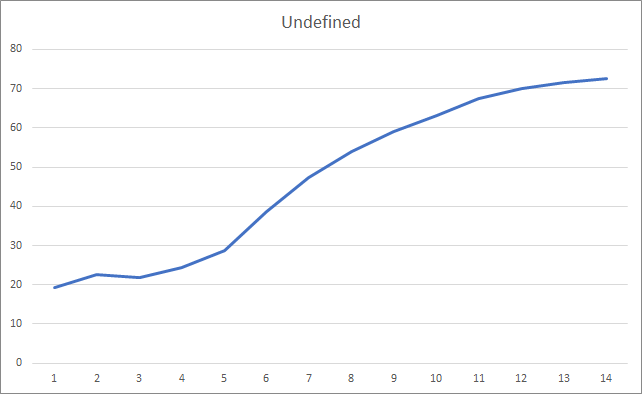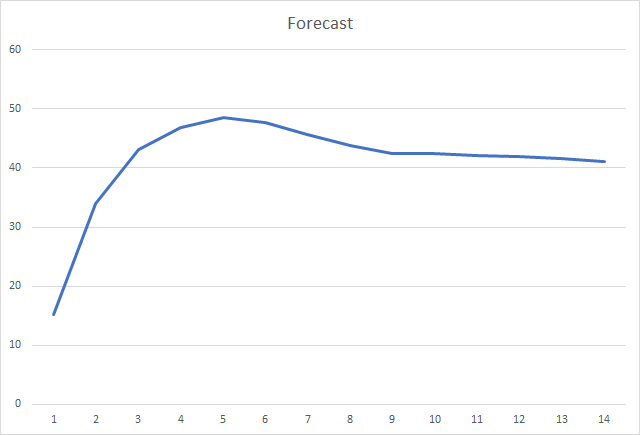### 本文中用到的程序

# 名称 类型 说明
1 Fractal_OCL_Adam.mq5  智能交易系统 一款采用 OpenCL 和 Adam 训练方法的分类神经网络 EA（输出层中有 3 个神经元）
2 NeuroNet.mqh 类库 用于创建神经网络的类库
3 NeuroNet.cl 代码库 OpenCL 程序代码库

MQL5.zip (430.54 KB)

#### 该作者的其他文章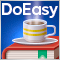DoEasy 函数库中的时间序列（第五十六部分）：自定义指标对象，从集合中的指标对象获取数据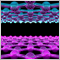市场及其全局模式中的物理学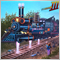模式搜索的暴力算法（第三部分）：新视野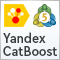基于暴力算法的 CatBoost 模型高级重采样与选择